StatLect

Sequences

Letbe a set of objects (e.g., real numbers, events, random variables). A sequence of elements ofis a function from the set of natural numbersto the set, i.e., a correspondence that associates one and only one element ofto each natural number. In other words, a sequence of elements ofis an ordered list of elements of, where the ordering is provided by the natural numbers.

A sequence is usually indicated by enclosing a generic element of the sequence in curly brackets:whereis the-th element of the sequence. Alternative notations are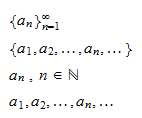Thus, ifis a sequence,is its first element,is its second element,is its-th element, and so on.

Example Define a sequenceby characterizing its-th elementas follows:is a sequence of rational numbers. The elements of the sequence are,,,and so on.

Example Define a sequenceby characterizing its-th elementas follows: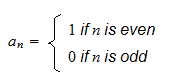is a sequence ofand. The elements of the sequence are,,,and so on.

Example Define a sequenceby characterizing its-th elementas follows: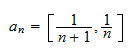is a sequence of closed subintervals of the interval. The elements of the sequence are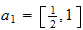,,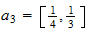,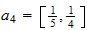and so on.Countable and uncountable sets

Letbe a set of objects.is a countable set if all its elements can be arranged into a sequence, i.e., if there exists a sequencesuch that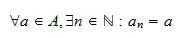In other words,is a countable set if there exists at least one sequencesuch that every element ofbelongs to the sequence.is an uncountable set if such a sequence does not exist. The most important example of an uncountable set is the set of real numbers.

Limit of a sequence

The concept of limit of a sequence is discussed in the lecture entitled Limit of a sequence.

The book

Most of the learning materials found on this website are now available in a traditional textbook format.

Glossary entries
Share